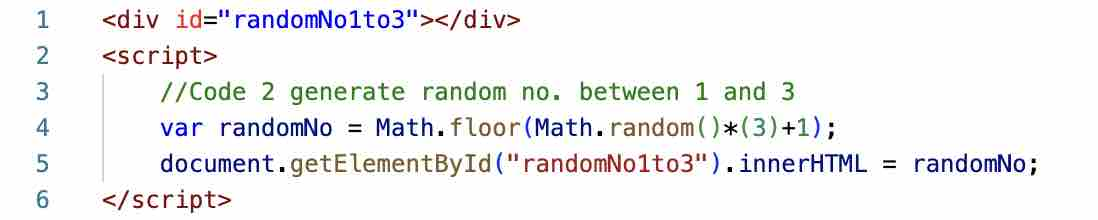# JavaScript: Generate Random Numbers between 1 and 3If you have a use-case to generate random numbers between 1 to 3, using JavaScript this can be achieved using the Math function,

Example:
``````<div id="randomNo1to3"></div>
<script>
//Code to generate random no. between 1 and 3
var randomNo = Math.floor(Math.random()*(3)+1);
document.getElementById("randomNo1to3").innerHTML = randomNo;
</script>``````

The output will be an of the number among [1,2,3]

-

Have Questions? Post them here!